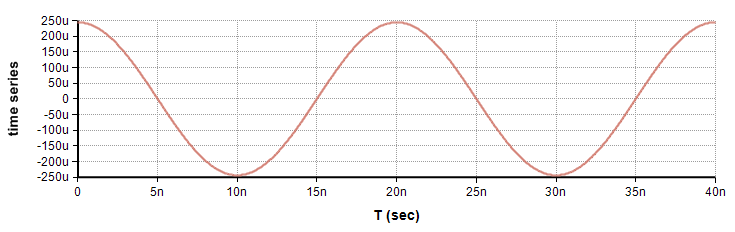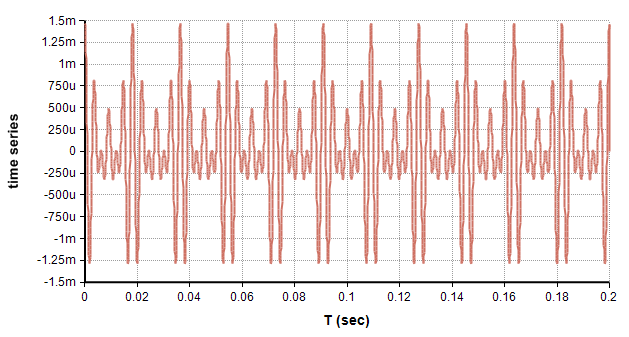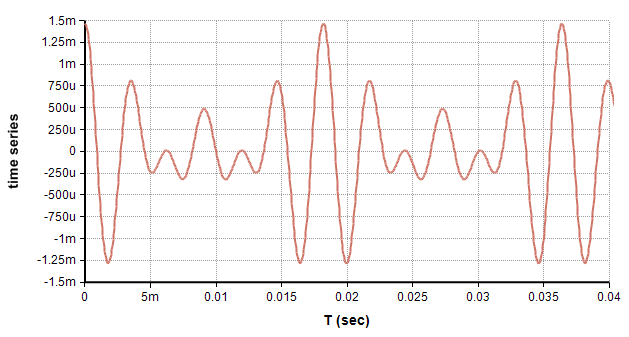# FFTInv

## FFTInv(x, Freq, T)

The Inverse Fast Fourier Transform (FFT) converts a frequency spectrum equally spaced values, «x», from the discrete frequency domain to the discrete time (or spatial) domain. «T» is the time index and «Freq» is the Frequency index, and these two indexes should have the same length. The frequency spectrum, «x», is indexed by «Freq» and contains complex numbers, encoding both the amplitude and phase at each frequency. The result is indexed by «T» and contains complex numbers in general. The units of «Freq» are cycles per «units of T».

The FFT function does the forward transformation from the time (or spatial) domain to the frequency domain.

## The Discrete Fourier Transform

FFTInv computes the inverse discrete Fourier transform (DFT) in an efficient manner. The inverse DFT is defined given by

$\displaystyle{ h_k = {1\over n} \sum_{i=0}^{n-1} x_i e^{-2j\pi i k/n} }$

where $\displaystyle{ j }$ is the imaginary number $\displaystyle{ \sqrt{-1} }$, and n is the number of points in «T» and «Freq». This is a discrete approximation to the continuous Fourier Transform given by

$\displaystyle{ h(t) = \int_{-\infty}^\infty x(f) e^{-2j \pi f t} df }$

### Units and spacing

Let $\displaystyle{ \Delta F }$ denote the spacing between points in «Freq» and $\displaystyle{ n }$ be the number of points. Then the spacing $\displaystyle{ \Delta t }$ of the points in «T» is $\displaystyle{ \Delta t = 1 / n \Delta F }$. The quantity $\displaystyle{ 1/\Delta t }$ is called the sampling frequency, which should be at least twice the smallest frequency that is present in the underlying continuous signal.

You will often want to encode «Freq» as having both positive and negative frequencies, even if the positive and negative spectra are symmetric. You'll want to take the part of the spectrum corresponding to negative frequencies and place them to the right of the positive spectrum, treating «Freq» as if it wraps around. When you do this, the first element of «Freq» encodes the DC component (0 Hz), the second element is $\displaystyle{ \Delta F }$, and the last element is $\displaystyle{ -\Delta F }$.

For example, suppose your frequency spectrum is sampled from 0 to 999.9Hz in increments of $\displaystyle{ \Delta F=0.1 Hz }$, for a total of 10K points. You would define «Freq» to be Concat(0..9999,-10K..-1)*0.1, and would map your positive-only spectrum (indexed by F) using x[@F = Mod(@Freq - 1, 10K, true) + 1] to obtain the symmetric spectrum with $\displaystyle{ n=20K }$ points Your spacing in the time domain will be $\displaystyle{ \Delta T=1 / (20K * 0.1)=0.5 millisecond }$. It would make sense to define your time-domain index, «T», as Sequence(0, 10-0.5m, 0.5m), or equivalently (0..20K-1)*0.5m.

## Considerations

Please see FFT for further information on the topics of Nyquist frequency, aliasing, mirroring, amplitude, phase, computational efficiency, and uses of the FFT.

## Examples

### Single frequency

The FFTInv of a single frequency is a cosine wave. In this example, we take the inverse FFT of a spectrum having a 50MHz component only. If we assume that the time-series is real-valued, then the spectrum must be symmetrical with a second component at -50MHz. We encode this an follows:

 Variable dF = 25M
Variable n = 4096
Variable dT = 1/(n*dF)
Index Freq = Concat(0..n/2-1, -n/2..-1)*dF
Index T = (0..n - 1)*dT
Variable x := Abs(Freq) = 50M
Variable time_series := RealPart(FFTInv(x, Freq, T))


The graph of time_series isAlthough the result is theoretically real-valued, the result from FFTInv includes a very small imaginary component. This arises from numeric round-off. Wrapping the result inside RealPart eliminates this.

## Major triad chord in music

A major chord in music contains the first, third and fifth notes of the major scale. The third note of a musical scale vibrates at a frequency 25% greater than the first note, and the fifth vibrates 50% faster. So, if you begin the A major scale at 220Hz, the third (C#) would be at 1.25*220 = 275Hz and the fifth (E) would be at 1.5*220 = 330Hz. In this example, we'll compute the vibration in time for an A-major musical chord.

 Variable dF = 25M
Variable n = 4096
Variable dT = 1/(n*dF)
Index Freq = [[Concat]](0..n/2-1,-n/2..-1)*dF
Index T = (0..n-1)*dT
Variable x := Abs(Freq) = 220 or Abs(Freq) = 275 or Abs(Freq) = 330
Variable time_series := RealPart(FFTInv(x, Freq, T))


The time series vibration isYou can use graph settings, manual axis scaling, to zoom into the first few cycles for more detail.## History

Introduced in Analytica 4.5.